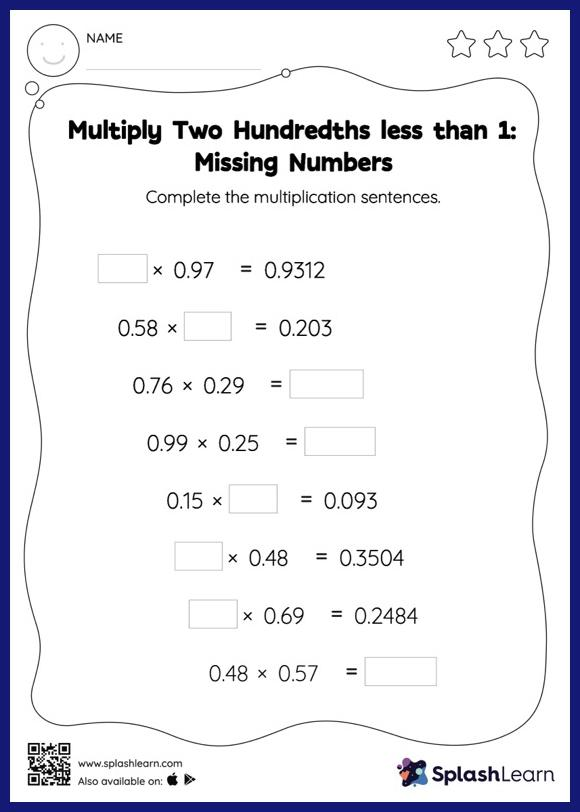# Multiply Two Hundredths less than 1: Missing Numbers Worksheet

Home > Multiply Two Hundredths less than 1: Missing NumbersStudents demonstrate their problem-solving ability as they work on this multiply two hundredths less than 1 worksheet. When students multiply two decimals, they do so without considering the decimal point at first. They find the product and add the decimal point at the appropriate place in it. This approach helps them in finding the missing number in decimal multiplication as with multiply two hundredths less than 1 worksheet. In each problem, the numbers are laid out in the horizontal format. Students should try to use different strategies involving composing and decomposing numbers to solve these problems. This will help them develop flexibility and fluency.